## Saturday, November 25, 2006

### Solution to the general cubic equation

Girolamo Cardano was able to find a general solution to the cubic equation based on Nicolo Fontana Tartaglia's solution to the depressed cubic equation.

For an equation of the form x3 + bx2 + cx + d = 0, Cardano did this by setting x = y - b/3.

The solution is complicated. Still, this solution was a very important step forward past the mathematics of Ancient Greece. Additionally, it would lead eventually to the acceptance of imaginary numbers and the establishment of group theory.

Lemma 1: Cardano's simplification of general cubic equation

If:

x3 + bx2 + cx + d = 0

Then there exists y,p,q such that:

y3 + py + q = 0

Proof:

(1) x3 + bx2 + cx + d = 0

(2) Let y = x + b/3

(3) Then, x = y - b/3

(4) x3 = (y - b/3)3 = y3 - 3by2/3 + 3b2y/9 - b3/27 =

= y3 - by2 + b2y/3 - b3/27

(5) bx2 = b(y - b/3)2 = by2 - 2b2y/3 + b3/9

(6) x3 + bx2 = y3 - b2y/3 + 2b3/27

(7) x3 + bx2 + cx + d =

= y3 - b2y/3 + 2b3/27 + c(y - b/3) + d =

= y3 + (c - b2/3)y + (2b3/27 - bc/3 + d)

(8) Let:

p = c - b2/3

q = 2b3/27 - bc/3 + d

QED

Theorem: Solution to General Cubic Equation

If:

ax3 + bx2 + cx + d = 0

Then: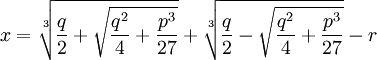where: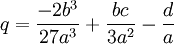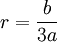Proof:

(1) ax3 + bx2 + cx + d = 0

(2) If we divide all sides by a, we get:

x3 + (b/a)x2 + (c/a)x + (d/a) = 0

(3) Then using Lemma 1 above, if we set:

y = x - (b/a)/3

p = (c/a) - (b/a)2/3

q = -2(b/a)3/27 + (b/a)(c/a)/3 - (d/a)

Then we have:

y3 + py - q = 0

or:

y3 + py = q

(4) Now, using the solution for the depressed cubic (see Theorem, here),

we have: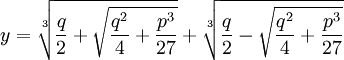(5) Finally, adjusting for step #3, if we let:

r = (b/a)/3

Then we have:QED

### Proof of Solution to Depressed Cubic

In today's blog, I will show a proof of the solution to the depressed cubic equation. Using Girolamo Cardano's trick (next blog), it is possible to use the solution to the depressed cubic equation to solve the general cubic equation.

Lemma 1: x3 = bx + c → ∃ u,v such that: b=3uv, c=u3 + v3, x = u + v

Proof:

(1) Let x3 = bx + c.

(2) Let x = u + v

(3) (u + v)3 = u3 + 3u2v + 3uv2 + v3 = 3uv(u + v) + (u3 + v3)

(4) 3uv(u + v) + (u3 + v3) = b(u + v) + c

(5) So that b = 3uv and c = u3 + v3

QED

Lemma 2: if b=3uv and c=u3 + v3, then:

we can assume:

u3 = c/2 + √(c/2)2 - (b/3)3

v3 = c/2 - √(c/2)2 - (b/3)3

Proof:

(1) b=3uv → v = b/3u

(2) u3 + v3 = u3 + (b/3u)3 =

= u3 + b3/(27u3) = c

(3) So that we have:

u3 + b3/(27u3) - c = 0

(4) Multiplying both sides by u3 gives us:

u6 + b3/27 - cu3 = 0

(5) Using the solution for quadratic equations (see here) gives us:

u3 = (c/2) ± √(c/2)2 - (b/3)3

(6) Since u3 + v3 = c, we can assume that:

u3 = (c/2) + √(c/2)2 - (b/3)3

v3 = (c/2) - √(c/2)2 - (b/3)3

QED

Theorem: Solution of the Depressed Cubic

if:

x3 + bx = c

then:Proof:

(1) x3 + bx = c

(2) So that we have:

x3 = (-b)x + c

(3) Using Lemma 1 above, we see that there exists u,v such that:

x = u + v

(-b) = 3uv

c = u3 + v3

(4) Using Lemma 2 above, we see that:

u3 = (c/2) + √(c/2)2 - (-b/3)3 = (c/2) + √(c/2)2 + (b/3)3

v3 = (c/2) - √(c/2)2 - (-b/3)3 = (c/2) - √(c/2)2 + (b/3)3

(5) Since x = u+v, we have:QED

## Friday, November 24, 2006

### Depressed Cubic

Scipione del Ferro's great discovery which eventually led to the general solution of the cubic equation was the solution of the depressed cubic equation:

x3 + bx = c

In today's blog, I will show how del Ferro solved this equation. The content is taken from Paul J. Nahin's The Story of √-1

(1) Let x = u+v

(2) Then:

x3 + bx = (u + v)3 + b(u + v) =

= u3 + 3u2v + 3uv2 + v3 + b(u + v) =

= u3 + v3 + (3uv + b)(u + v) = c

(3) Now, if:

(3uv + b) = 0

then:

u3 + v3 = c

(4) Now,

(3uv + b) = 0 → 3uv = -b

and further:

v = -b/(3u)

(5) So if:

u3 + v3 = c, then:

u3 + [-b/(3u)]3 - c =

u3 + (-b3)/(27u3) - c =

u6 + (-b3/27 - cu3 = 0

(6) But this is an example of a quadratic equation since if:

z = u3, then we have:

u6 + (-b3/27) - cu3 =

= z2 - cz -(b3/27)

(7) Using the solution for the quadratic equation (see here), we have:

z = (c/2) ± √c2 - 4(-b3/27)/2 =

=(c/2) ± √c2/4 + b3/27 = u3

(8) Considering only the positive form, we have: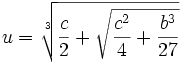(9) Since v3 = c - u3, we have:

v3 = c - (c/2 + √c2/4 + b3/27 =

= c/2 - √c2/4 + b3/27

(10) This then gives us: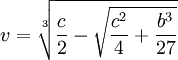(11) Since x = u + v, this gives us that:(12) Since (-1)3 = (-1)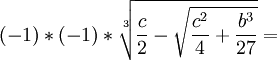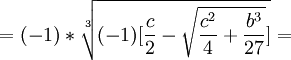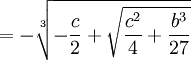(13) This tells us that we can restate (11) as the following: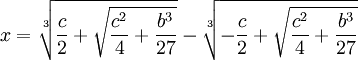In another blog, I present a proof that this really is the solution of the depressed cubic.

References

## Wednesday, November 22, 2006

### Nicolo Fontana Tartaglia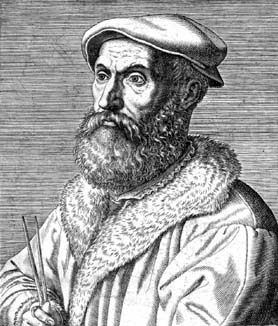Nicolo Fontana "Tartaglia" was born around 1500 in Venice. His father was a "mail rider", a person who would ride from town to town, to make deliveries. When Tartaglia was six years old, his father was murdered during one of these trips.

When Tartaglia was 12, the French took over his town of Brescia. There had been fierce fighting and in retaliation, there was a great slaughter of the Brescian townfolks. It is said that 46,000 inhabitants were killed in the fighting and the revenge killings. Tartaglia, his mother, and his sister hid away in a cathedral. In the course of the fighting, Tartaglia was attacked and left for dead.

Somehow, he survived but for the rest of his life, he bore terrible scars near his jaw and had difficulty speaking. It was in this way that he got his nickname "Tartaglia" which means "the stammerer". As an adult, he would always wear a beard in order to hide the scars.

Tartaglia took an interest in mathematics and demonstrated strong ability which earned him the patronage of Ludovico Balbisonio who paid for him to attend the University of Padua. By 1516, he had moved to Verona where he made his living by lecturing on mathematics.

In 1534, Tartaglia was working as a math teacher in Venice. While this was still a low paying position, he began to develop a reputation by participating in public mathematical challenges.

In 1535, there was a famous math contest between Tartaglia and Fior. Fior was a student of Scipione del Ferro who had already solved the depressed cubic equation and had given the solution to Fior before he died. Tartaglia had by this time found a solution to one form of the cubic equation which was not directly solvable by the depressed cubic equation. Both Tartaglia and Fior submitted 30 problems that the other had to solve. Tartaglia could tell that Fior had a solution to the depressed cubic so he worked hard to find it. On February 13, 1535, Tartaglia solved the depressed cubic. Tartaglia easily solved all 30 of Fior's problems and won the contest.

Girolamo Cardano heard about Tartaglia's solution of the depressed cubic. Cardano, by this time, had a reputation as a brilliant and well-connected mathematician and physician. He approached Tartaglia with the hope of including Tartaglia's result in a book he was working on. Tartaglia declined.

Cardano promised to provide a letter of introduction to a very influential patron in Milan and invited Tartaglia to his home. Tartaglia, who still held his teacher's job, accepted Cardano's invitation to visit. While there, Cardano was able to convince Tartaglia to reveal his method for solving the depressed cubic. Cardano promised that he would not publish the solution without getting permission from Tartaglia.

Using Tartaglia's method, Cardano was able to solve the general cubic equation and his assistant Lodovico Ferrari was able to solve the general quartic equation. Tartaglia refused to give Cardano permission to publish the method. It is probable that Tartaglia believed that he could use his method to continue to win math challenges.

When Cardano learned that Scipione del Ferro was the first to solve the depressed cubic, he decided to go ahead and publish his book Ars Magna even without Tartaglia's permission. In the book, he gives Tartaglia and del Ferro credit for the solving the depressed cubic. He then shows how the general cubic equation can be reduced to a form of the depressed cubic.

This publishing of Cardano's book infuriated Tartaglia who proceeded to publish his own book called New Problems and Inventions. In it, he attacked Cardano. Despite this, Cardano's book was a bestseller and established Cardano as the leading mathematician of his age.

Tartaglia challenged Cardano to a public math debate but Cardano refused. Cardano's assistant Ferrari offered to take up the challenge in Cardano's place. At this time, Ferrari was a relatively unknown mathematician and Tartaglia had little to gain from the challenge. On the other hand, if he had succeeded in challenging Cardano, because of Cardano's reputation, the debate could have helped his mathematical standing.

Tartaglia was offered a very lucrative post that he could get if he defeated Ferrari in the challenge. The math debate between Tartaglia and Ferrari occurred on August 10, 1548. Ferrari had clearly mastered the general cubic and quartic equations and it was very clear that Tartaglia would not be able to answer all of Ferrari's problems. Rather than admit this, Tartaglia left before the challenge completed.

Having lost the challenge, Tartaglia found that his existing position was threatened. In this way, Tartaglia saw his reputation collapse. It is said that for the rest of his life, he harbored resentment against Cardano. It is also said that he died in poverty. Tartaglia died on December 13, 1557.

References

### Scipione del Ferro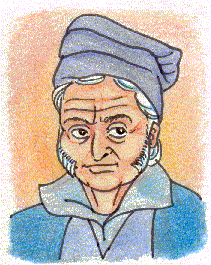Scipione del Ferro was the first mathematician to find a solution to the depressed cubic equation which is the equation of the form:

ax3 + bx = c

Gerolamo Cardano would later show how the solution to the depressed cubic equation leads to the solutions of the general cubic equation.

Del Ferro was born on February 6, 1465 in Bologna, Italy. His father was a papermaker which was a profession that was growing in importance due the invention of the printing with moveable types (this was invented by Johannes Gutenberg in 1447). Not much is known about del Ferro's early life but it is believed that he attended University of Bologna which had been founded in the eleventh century.

In 1496, del Ferro became a lecturer in mathematics at the University of Bologna. If del Ferro wrote any books, none of them survive. It is believed that he was very secretive about his discoveries and communicated them only with close friends and selected students. He kept his discoveries in a notebook. This notebook would later become an important record in establishing that del Ferro had indeed discovered the solution to the depressed cubic equation.

There is a story often told that del Ferro kept his solution to the depressed cubic equation secret until on his deathbed, he revealed it. According to the MacTutor biography, this story is false. There are numerous people who had knowledge of del Ferro's solution.

Del Ferro is believed to have died on November 5, 1526. On his death, his notebook was given to his son-in-law Hannibal Nave. It was discovery of this notebook that motivated Cardano to release his famous math book Ars Magna. Cardano had promised Tartaglia that he would not reveal the method but he felt that del Ferro's earlier discovery of the method excused him from this promise.

Today, with the notebook lost, it is very difficult to assess the mathematical contributions of del Ferro. There is no doubt that he was the first to solve a very difficult problem which would later give birth to imaginary numbers and group theory.

Cardano would later write (quoted from MacTutor biography on del Ferro):

Scipione Ferro of Bologna, almost thirty years ago, discovered the solution of the cube and things equal to a number [which in today's notation is the case x3+ mx = n], a really beautiful and admirable accomplishment. In distinction this discovery surpasses all mortal ingenuity, and all human subtlety. It is truly a gift from heaven, although at the same time a proof of the power of reason, and so illustrious that whoever attains it may believe himself capable of solving any problem.

References